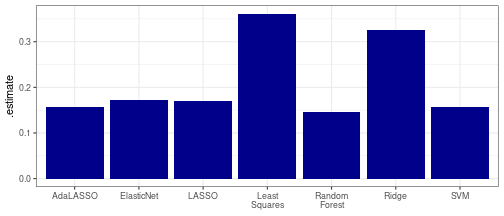# Multinomial Classification

``````library(dplyr); library(tidyr); library(purrr) # Data wrangling
library(ggplot2); library(stringr) # Plotting
library(tidyfit)   # Auto-ML modeling
``````

Multinomial classification is possible in `tidyfit` using the methods powered by `glmnet`, `e1071` and `randomForest` (LASSO, Ridge, ElasticNet, AdaLASSO, SVM and Random Forest). Currently, none of the other methods support multinomial classification.^[Feature selection methods such as `relief` or `chisq` can be used with multinomial response variables. I may also add support for multinomial classification with `mboost` in future.] When the response variable contains more than 2 classes, `classify` automatically uses a multinomial response for the above-mentioned methods.

Here’s an example using the built-in `iris` dataset:

``````data("iris")

# For reproducibility
set.seed(42)
ix_tst <- sample(1:nrow(iris), round(nrow(iris)*0.2))

data_trn <- iris[-ix_tst,]
data_tst <- iris[ix_tst,]

as_tibble(iris)
#> # A tibble: 150 × 5
#>    Sepal.Length Sepal.Width Petal.Length Petal.Width Species
#>           <dbl>       <dbl>        <dbl>       <dbl> <fct>
#>  1          5.1         3.5          1.4         0.2 setosa
#>  2          4.9         3            1.4         0.2 setosa
#>  3          4.7         3.2          1.3         0.2 setosa
#>  4          4.6         3.1          1.5         0.2 setosa
#>  5          5           3.6          1.4         0.2 setosa
#>  6          5.4         3.9          1.7         0.4 setosa
#>  7          4.6         3.4          1.4         0.3 setosa
#>  8          5           3.4          1.5         0.2 setosa
#>  9          4.4         2.9          1.4         0.2 setosa
#> 10          4.9         3.1          1.5         0.1 setosa
#> # ℹ 140 more rows
``````

## Penalized classification algorithms to predict `Species`

The code chunk below fits the above mentioned algorithms on the training split, using a 10-fold cross validation to select optimal penalties. We then obtain out-of-sample predictions using `predict`. Unlike binomial classification, the `fit` and `pred` objects contain a `class` column with separate coefficients and predictions for each class. The predictions sum to one across classes:

``````fit <- data_trn %>%
classify(Species ~ .,
LASSO = m("lasso"),
Ridge = m("ridge"),
ElasticNet = m("enet"),
SVM = m("svm"),
`Random Forest` = m("rf"),
`Least Squares` = m("ridge", lambda = 1e-5),
.cv = "vfold_cv")

pred <- fit %>%
predict(data_tst)
``````

Note that we can add unregularized least squares estimates by setting `lambda = 0` (or very close to zero).

Next, we can use `yardstick` to calculate the log loss accuracy metric and compare the performance of the different models:

``````metrics <- pred %>%
group_by(model, class) %>%
mutate(row_n = row_number()) %>%The least squares estimate performs poorest, while the random forest (nonlinear) and the support vector machine (SVM) achieve the best results. The SVM is estimated with a linear kernel by default (use `kernel = <chosen_kernel>` to use a different kernel).